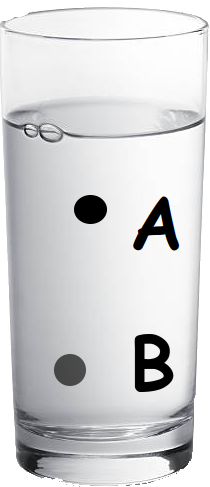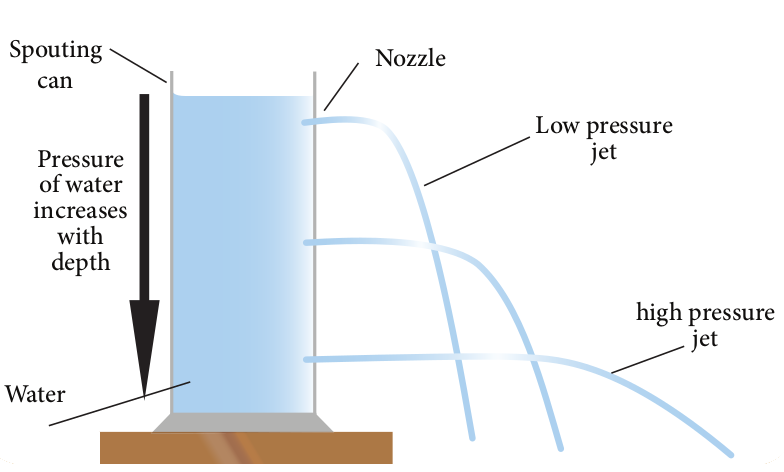Force and Pressure - Different forces applied by liquid

Buoyancy Force, Viscous Force, Surface Tension and Pascal's Law.

Learner's Guide

Motivation:

In this session, we will learn about different forces exerted by liquid:

• Buoyancy Force
• Surface Tension
• Viscous Force
• Pascal's Law

We will also learn about reaction of liquid when force is applied by understanding Pascal's Law.1. Pressure at A is more compared to B
2. Pressure at A and B are same
3. Pressure at B is more compared to A
4. Can not comment.

Weight of water at A is because by water above point.

Weight of water at B is because of water above B.

We know that pressure exerted by liquids are because of their weight.

So pressure is more at point B than A.

If you make holes at A and B points, water will come out with stronger jet.Buoyancy Force:

Buoyancy force acts only when low density object is dipped in a liquid.

True or False?

True.

For an object which has higher density than liquid do not have any buoyancy force acting on it.

Surface Tension:

A boat floats on water because of ___________________

1. surface tension
2. buoyancy force
3. viscous force
4. All

Buoyancy Force

Boats are made from material which are less denser compared to water.

Generally wood is used in base part of boat which is less denser than water.Pascal's Law:

1/12Can we say that using pascal's law, we can transfer force without loosing it's effectiveness.

Effectiveness of force is nothing but pressure.

According to pascal's law, pressure exerted at any point of liquid is experienced at all other locations of liquid.

Viscous Force:

Which of the following a contact force?

1. Surface Tension
2. Buoyancy Force
3. Viscous Force
4. All

All

Exercise

Tip: Think before answering.

• Which force is responsible for mosquito to sit on water surface?

1. Buoyancy Force
2. Surface tension
3. Pascal's law
4. Viscous Force
Difficulty Level: Easy

Surface tension

• Surface of water or a liquid has more molecule compare to deep regions.

True or False?

Difficulty Level: Easy

True.

• Which one will consume less energy?

1. Driving boat on oil
2. Driving boat on water
3. Both will consume same energy
Difficulty Level: Moderate

Driving boat on water will consume less energy as it has less viscosity compared to oil.

• Water form drops because of __________________

1. Buoyancy Force
2. Surface tension
3. Viscous force
4. Pascal's law
Difficulty Level: Moderate

Surface tension